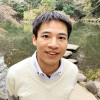場の量子論セミナーセミナー

Localization and universality in non-Hermitian many-body systems

2020年5月29日15:00 - 16:30

Recent study on isolated quantum many-body systems have revealed two different phases distinguished by their dynamics and spectral statistics. One is an ergodic phase whose spectral statistics exhibit universality of random matrices, and the other is a many-body localized phase where dynamics is constrained due to strong disorder. In this talk, we show that novel and rich physics concerning such localization and universality appears in non-Hermitian many-body systems, which have been utilized in diverse scientific disciplines from open quantum systems to biology. As a first topic, we analyze non-Hermitian quantum many-body systems in the presence of interaction and disorder . We demonstrate that a novel real-complex transition occurs upon many-body localization of non-Hermitian interacting systems with asymmetric hopping that respect time-reversal symmetry. As a second topic, we show that “Dyson’s threefold way,” a threefold symmetry classification of universal spectral statistics of random matrices, is nontrivially extended to non-Hermitian random matrices . We report our discovery of two distinct universality classes characterized by transposition symmetry, which is distinct from time-reversal symmetry due to non-Hermiticity. We show that the newly found universality classes indeed manifest themselves in dissipative quantum many-body ergodic systems described by Lindblad equations.

イベント公式言語: 英語セミナー

Gradient Flow Equation and Its Applications

2020年5月15日13:30 - 15:00

Gradient flow is the one of the methods to suppress the ultraviolet divergence in gauge theories. The any correlation functions in terms of the flowed field, which is defined by the gradient flow equation, are finite without additional renormalizations. Because of this surprising property, the methods has been studied widely, especially in the lattice field theory. In this seminar, we introduce what the gradient flow is briefly. And we show our work, “generalized gradient flow equation”, which is the gradient flow equation for field theories with nonlinearly realized symmetry. Applying the formalism to a supersymmetric theory and O(N) non linear sigma model, we obtain the SUSY gradient flow and the Large N gradient flow. We also refer to the current research, the gradient flow of the supersymmetric theory with the non-renormalization theorem and the new formalism to obtain the sphalerons, which is one of the static classical solutions, using gradient flow methods, if time allows.

イベント公式言語: 英語